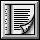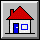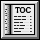ELKS 2001 STRING Class`indexing`
```description: "Sequences of characters, accessible through %
%integer indices in a contiguous range starting %
%from one."```
`class interface`
`STRING`
`creation `
```make_empty
-- Create empty string.
ensure
empty: count = 0```
```make_filled (c: CHARACTER; n: INTEGER)
-- Create string of length n filled with c.
require
valid_count: n >= 0
ensure
count_set: count = n
filled: occurrences (c) = count```
```make (suggested_capacity: INTEGER)
-- Create empty string, or remove all characters from
-- existing string.
require
non_negative_suggested_capacity: suggested_capacity >= 0
ensure
empty_string: count = 0```
```make_from_string (s: STRING)
-- Initialize from the character sequence of s.
require
s_not_void: s /= Void
ensure
initialized: same_string (s)```
`feature -- Initialization`
```make (suggested_capacity: INTEGER)
-- Create empty string, or remove all characters from
-- existing string.
require
non_negative_suggested_capacity: suggested_capacity >= 0
ensure
empty_string: count = 0```
```from_c (c_string: POINTER)
-- Set the current STRING from a copy of the
-- zero-byte-terminated memory starting at `c_string'.
require
c_string_exists: c_string /= default_pointer
ensure
no_zero_byte: not has ('%/0/')
characters: -- for all i in 1..count, item(i) equals the
-- ASCII character at address c_string+i-1
correct_count: -- the ASCII character at address
-- c_string+count is NUL```
```make_from_string (s: STRING)
-- Initialize from the character sequence of s.
require
s_not_void: s /= Void
ensure
initialized: same_string (s)```
`feature -- Basic specifiers`
```count: INTEGER
-- Number of characters```
```item (i: INTEGER): CHARACTER
-- Character at index i
require
valid_index: valid_index (i)```
`feature -- Access`
```hash_code: INTEGER
-- Hash code value (vendor dependent hashing function)
-- (From HASHABLE.)
ensure
good_hash_value: Result >= 0```
```index_of (c: CHARACTER; start_index: INTEGER): INTEGER
-- Index of first occurrence of c at or after start_index;
-- 0 if none
require
valid_start_index: start_index >= 1 and start_index <= count + 1
ensure
valid_result: Result = 0 or (start_index <= Result and Result <= count)
zero_if_absent: (Result = 0) = not substring (start_index, count).has (c)
found_if_present: substring (start_index, count).has (c) implies
item (Result) = c
none_before: substring (start_index, count).has (c) implies
not substring (start_index, Result - 1).has (c)```
```string: STRING
-- New STRING having the same character sequence as Current
ensure
string_not_void: Result /= Void
string_type: Result.same_type ("")
first_item: count > 0 implies Result.item (1) = item (1)
recurse: count > 1 implies
Result.substring (2, count).is_equal (substring (2, count).string)```
```substring (start_index, end_index: INTEGER): like Current
-- New object containing all characters
-- from start_index to end_index inclusive
require
valid_start_index: 1 <= start_index
valid_end_index: end_index <= count
meaningful_interval: start_index <= end_index + 1
ensure
substring_not_void: Result /= Void
substring_count: Result.count = end_index - start_index + 1
first_item: Result.count > 0 implies Result.item (1) = item (start_index)
recurse: Result.count > 0 implies
Result.substring (2, Result.count).is_equal
(substring (start_index + 1, end_index))```
```substring_index (other: STRING; start_index: INTEGER): INTEGER
-- Index of first occurrence of other at or after start_index;
-- 0 if none
require
other_not_void: other /= Void
valid_start_index: start_index >= 1 and start_index <= count + 1
ensure
valid_result: Result = 0 or else
(start_index <= Result and Result <= count - other.count + 1)
zero_if_absent: (Result = 0) =
not substring (start_index, count).has_substring (other)
at_this_index: Result >= start_index implies
other.same_string (substring (Result, Result + other.count - 1))
none_before: Result > start_index implies
not substring (start_index, Result + other.count - 2).has_substring (other)```
```infix "@" (i: INTEGER): CHARACTER
-- Character at index i
require
valid_index: valid_index (i)
ensure
definition: Result = item (i)```
`feature -- Measurement`
```occurrences (c: CHARACTER): INTEGER
-- Number of times c appears in the string
ensure
zero_if_empty: count = 0 implies Result = 0
recurse_if_not_found_at_first_position:
(count > 0 and then item (1) /= c) implies
Result = substring (2, count).occurrences (c)
recurse_if_found_at_first_position:
(count > 0 and then item (1) = c) implies
Result = 1 + substring (2, count).occurrences (c)```
`feature -- Comparison`
```is_equal (other: like Current): BOOLEAN
-- Is other attached to an object considered equal
-- to current object?
-- (Redefined from GENERAL.)
require
other_not_void: other /= Void
ensure
consistent: standard_is_equal (other) implies Result
same_type: Result implies same_type (other)
symmetric: Result implies other.is_equal (Current)
definition: Result = (same_type (other) and then
count = other.count and then
(count > 0 implies (item (1) = other.item (1) and
substring (2, count).is_equal (other.substring (2, count)))))```
```same_string (other: STRING): BOOLEAN
-- Do Current and other have the same character sequence?
require
other_not_void: other /= Void
ensure
definition: Result = string.is_equal (other.string)```
```infix "<" (other: STRING): BOOLEAN
-- Is string lexicographically lower than other?
-- (From COMPARABLE.)
require
other_exists: other /= Void
ensure
asymmetric: Result implies not (other < Current)
definition: Result = (count = 0 and other.count > 0 or
count > 0 and then other.count > 0 and then
(item (1) < other.item (1) or
item (1) = other.item (1) and
substring (2, count) < other.substring (2, other.count)))```
```infix "<=" (other: like Current): BOOLEAN
-- Is current object less than or equal to other?
-- (From COMPARABLE.)
require
other_exists: other /= Void
ensure
definition: Result = (Current < other or is_equal (other))```
```infix ">=" (other: like Current): BOOLEAN
-- Is current object greater than or equal to other?
-- (From COMPARABLE.)
require
other_exists: other /= Void
ensure
definition: Result = (other <= Current)```
```infix ">" (other: like Current): BOOLEAN
-- Is current object greater than other?
-- (From COMPARABLE.)
require
other_exists: other /= Void
ensure
definition: Result = (other < Current)```
```max (other: like Current): like Current
-- The greater of current object and other
-- (From COMPARABLE.)
require
other_exists: other /= Void
ensure
current_if_not_smaller: (Current >= other) implies (Result = Current)
other_if_smaller: (Current < other) implies (Result = other)```
```min (other: like Current): like Current
-- The smaller of current object and other
-- (From COMPARABLE.)
require
other_exists: other /= Void
ensure
current_if_not_greater: (Current <= other) implies (Result = Current)
other_if_greater: (Current > other) implies (Result = other)```
```three_way_comparison (other: like Current): INTEGER
-- If current object equal to other, 0; if smaller,
-- -1; if greater, 1.
-- (From COMPARABLE.)
require
other_exists: other /= Void
ensure
equal_zero: (Result = 0) = is_equal (other)
smaller: (Result = -1) = Current < other
greater_positive: (Result = 1) = Current > other```
`feature -- Status report`
```has (c: CHARACTER): BOOLEAN
-- Does Current contain c?
ensure
false_if_empty: count = 0 implies not Result
true_if_first: count > 0 and then item (1) = c implies Result
recurse: (count > 0 and then item (1) /= c) implies
(Result = substring (2, count).has (c))```
```has_substring (other: STRING): BOOLEAN
-- Does Current contain other?
require
other_not_void: other /= Void
ensure
false_if_too_small: count < other.count implies not Result
true_if_initial: (count >= other.count and then
other.same_string (substring (1, other.count)))
implies Result
recurse: (count >= other.count and then
not other.same_string (substring (1, other.count))) implies
(Result = substring (2, count).has_substring (other))```
```is_boolean: BOOLEAN
-- Does Current represent a BOOLEAN?
ensure
is_boolean: Result = (same_string ("true") or same_string ("false"))```
```is_double: BOOLEAN
-- Does Current represent a DOUBLE?
ensure
syntax_and_range:
-- Result is true if and only if the following two
-- conditions are satisfied:
--
-- 1. In the following BNF grammar, the value of
--    Current can be produced by "Real_literal":
--
-- Real_literal    = Mantissa [Exponent_part]
-- Exponent_part   = "E" Exponent
--                 | "e" Exponent
-- Exponent        = Integer_literal
-- Mantissa        = Decimal_literal
-- Decimal_literal = Integer_literal ["." Integer]
-- Integer_literal = [Sign] Integer
-- Sign            = "+" | "-"
-- Integer         = Digit | Digit Integer
-- Digit           = "0"|"1"|"2"|"3"|"4"|"5"|"6"|"7"|"8"|"9"
--
-- 2. The numerical value represented by Current
--    is within the range that can be represented
--    by an instance of type DOUBLE.```
```is_empty: BOOLEAN
-- Is string empty?
ensure
definition: Result = (count = 0)```
```is_integer: BOOLEAN
-- Does Current represent an INTEGER?
ensure
syntax_and_range:
-- Result is true if and only if the following two
-- conditions are satisfied:
--
-- 1. In the following BNF grammar, the value of
--    Current can be produced by "Integer_literal":
--
-- Integer_literal = [Sign] Integer
-- Sign            = "+" | "-"
-- Integer         = Digit | Digit Integer
-- Digit           = "0"|"1"|"2"|"3"|"4"|"5"|"6"|"7"|"8"|"9"
--
-- 2. The integer value represented by Current
--    is within the (inclusive) range
--    minimum_integer..maximum_integer
--    where `minimum_integer' and `maximum_integer'
--    are the constants defined in class PLATFORM.```
```is_real: BOOLEAN
-- Does Current represent a REAL?
ensure
syntax_and_range:
-- Result is true if and only if the following two
-- conditions are satisfied:
--
-- 1. In the following BNF grammar, the value of
--    Current can be produced by "Real_literal":
--
-- Real_literal    = Mantissa [Exponent_part]
-- Exponent_part   = "E" Exponent
--                 | "e" Exponent
-- Exponent        = Integer_literal
-- Mantissa        = Decimal_literal
-- Decimal_literal = Integer_literal ["." Integer]
-- Integer_literal = [Sign] Integer
-- Sign            = "+" | "-"
-- Integer         = Digit | Digit Integer
-- Digit           = "0"|"1"|"2"|"3"|"4"|"5"|"6"|"7"|"8"|"9"
--
-- 2. The numerical value represented by Current
--    is within the range that can be represented
--    by an instance of type REAL.```
```valid_index (i: INTEGER): BOOLEAN
-- Is i within the bounds of the string?
ensure
definition: Result = (1 <= i and i <= count)```
`feature -- Element change`
```append_character (c: CHARACTER)
-- Append c at end.
ensure
new_count: count = old count + 1
appended: item (count) = c
stable_before: substring (1, count - 1).is_equal (old clone (Current))```
```append_string (s: STRING)
-- Append a copy of s at end.
require
s_not_void: s /= Void
ensure
appended: is_equal (old clone (Current) + old clone (s))```
```fill_with (c: CHARACTER)
-- Replace every character with c.
ensure
same_count: old count = count
filled: occurrences (c) = count```
```insert_character (c: CHARACTER; i: INTEGER)
-- Insert c at index i, shifting characters between
-- ranks i and count rightwards.
require
valid_insertion_index: 1 <= i and i <= count + 1
ensure
one_more_character: count = old count + 1
inserted: item (i) = c
stable_before_i: substring (1, i - 1).is_equal (old substring (1, i - 1))
stable_after_i: substring (i + 1, count).is_equal (old substring (i, count))```
```insert_string (s: STRING; i: INTEGER)
-- Insert s at index i, shifting characters between ranks
-- i and count rightwards.
require
string_not_void: s /= Void
valid_insertion_index: 1 <= i and i <= count + 1
ensure
inserted: is_equal (old substring (1, i - 1)
+ old clone (s) + old substring (i, count))```
```put (c: CHARACTER; i: INTEGER)
-- Replace character at index i by c.
require
valid_index: valid_index (i)
ensure
stable_count: count = old count
replaced: item (i) = c
stable_before_i: substring (1, i - 1).is_equal (old substring (1, i - 1))
stable_after_i: substring (i + 1, count).is_equal (old substring (i + 1, count))```
```replace_substring (s: STRING; start_index, end_index: INTEGER)
-- Replace the substring from start_index to end_index,
-- inclusive, with s.
require
string_not_void: s /= Void
valid_start_index: 1 <= start_index
valid_end_index: end_index <= count
meaningful_interval: start_index <= end_index + 1
ensure
replaced: is_equal (old (substring (1, start_index - 1) +
s + substring (end_index + 1, count)))```
`feature -- Removal`
```keep_head (n: INTEGER)
-- Remove all the characters except for the first n;
-- if n > count, do nothing.
require
n_non_negative: n >= 0
ensure
kept: is_equal (old substring (1, n.min (count)))```
```keep_tail (n: INTEGER)
-- Remove all the characters except for the last n;
-- if n > count, do nothing.
require
n_non_negative: n >= 0
ensure
kept: is_equal (old substring (count - n.min (count) + 1, count))```
```remove (i: INTEGER)
-- Remove i-th character, shifting characters between
-- ranks i + 1 and count leftwards.
require
valid_removal_index: valid_index (i)
ensure
removed: is_equal (old substring (1, i - 1) +
old substring (i + 1, count))```
```remove_head (n: INTEGER)
-- Remove the first n characters;
-- if n > count, remove all.
require
n_non_negative: n >= 0
ensure
removed: is_equal (old substring (n.min (count) + 1, count))```
```remove_substring (start_index, end_index: INTEGER)
-- Remove all characters from start_index
-- to end_index inclusive.
require
valid_start_index: 1 <= start_index
valid_end_index: end_index <= count
meaningful_interval: start_index <= end_index + 1
ensure
removed: is_equal (old (substring (1, start_index - 1) +
old (substring (end_index + 1, count))```
```remove_tail (n: INTEGER)
-- Remove the last n characters;
-- if n > count, remove all.
require
n_non_negative: n >= 0
ensure
removed: is_equal (old substring (1, count - n.min (count)))```
```wipe_out
-- Remove all characters.
ensure
empty_string: count = 0```
`feature -- Conversion`
```as_lower: like Current
-- New object with all letters in lower case
ensure
length: Result.count = count
anchor: count > 0 implies Result.item (1) = item (1).as_lower
recurse: count > 1 implies
Result.substring (2, count).is_equal (substring (2, count).as_lower)```
```as_upper: like Current
-- New object with all letters in upper case
ensure
length: Result.count = count
anchor: count > 0 implies Result.item (1) = item (1).as_upper
recurse: count > 1 implies
Result.substring (2, count).is_equal (substring (2, count).as_upper)```
```to_boolean: BOOLEAN
-- Boolean value;
-- "true" yields true, "false" yields false
require
represents_a_boolean: is_boolean
ensure
to_boolean: Result = same_string ("true")```
```to_double: DOUBLE
-- "Double" value; for example, when applied to "123.0",
-- will yield 123.0 (double)
require
represents_a_double: is_double ```
```to_integer: INTEGER
-- Integer value;
-- for example, when applied to "123", will yield 123
require
represents_an_integer: is_integer
ensure
single_digit: count = 1 implies
Result = ("0123456789").index_of (item (1), 1) - 1
minus_sign_followed_by_single_digit:
count = 2 and item (1) = '-' implies
Result = - substring (2, 2).to_integer
plus_sign_followed_by_single_digit:
count = 2 and item (1) = '+' implies
Result = substring (2, 2).to_integer
recurse_to_reduce_length:
count > 2 or count = 2 and not (("+-").has (item (1))) implies
Result // 10 = substring (1, count - 1).to_integer and
(Result \\ 10).abs = substring (count, count).to_integer```
```to_lower
-- Convert all letters to lower case.
ensure
length_and_content: is_equal (old as_lower)```
```to_real: REAL
-- Real value;
-- for example, when applied to "123.0", will yield 123.0
require
represents_a_real: is_real```
```to_upper
-- Convert all letters to upper case.
ensure
length_and_content: is_equal (old as_upper)```
`feature -- Duplication`
```copy (other: like Current)
-- Reinitialize by copying the characters of other.
-- (This is also used by clone.)
-- (From GENERAL.)
require
other_not_void: other /= Void
type_identity: same_type (other)
ensure
is_equal: is_equal (other)```
```infix "+" (other: STRING): like Current
-- New object which is a clone of `Current' extended
-- by the characters of `other'.
require
other_exists: other /= Void
ensure
result_not_void: Result /= Void
result_count: Result.count = count + other.count
initial: Result.substring (1, count).is_equal (Current)
final: Result.substring (count + 1, count + other.count).same_string (other)```
`feature -- Output`
```out: STRING
-- New STRING containing terse printable representation
-- of current object
-- (From GENERAL.)
ensure
out_not_void: Result /= Void
same_items: same_type ("") implies Result.same_string (Current)```
`invariant`
```irreflexive_comparison: not (Current < Current)
-- (From COMPARABLE.)```
`non_negative_count: count >= 0`
`end`
```--   The correctness of this specification is based on the following
--   assumptions:
--
--   1. No feature of STRING calls a command on any of its arguments.
--
--   2. No query of STRING changes the value of any basic specifier.
--
--   3. No feature of STRING shares internal structures in any way
--       that could lead to behaviour not deducible from this
--       specification.
--
--   4. The phrase "new STRING" in a header comment means a
--       newly-created STRING to which there is no reference other than
--       from `Result'.
--
--   5. The phrase "new object" in a header comment means a
--       newly-created object of type "like Current" to which
--       there is no reference other than from `Result'.```

 Copyright © 2001, Nonprofit International Consortium for Eiffel Last Updated: 28 December 2001